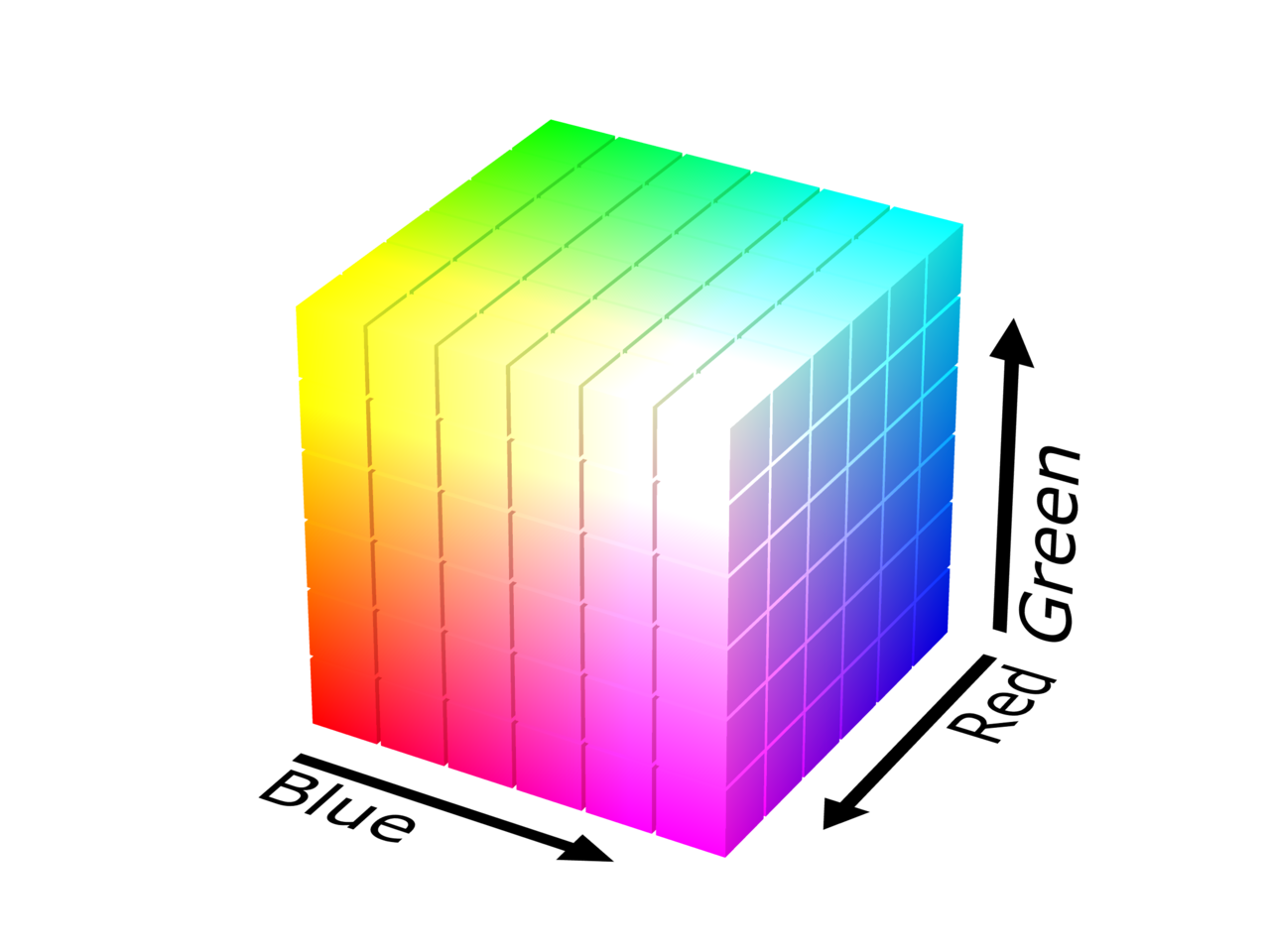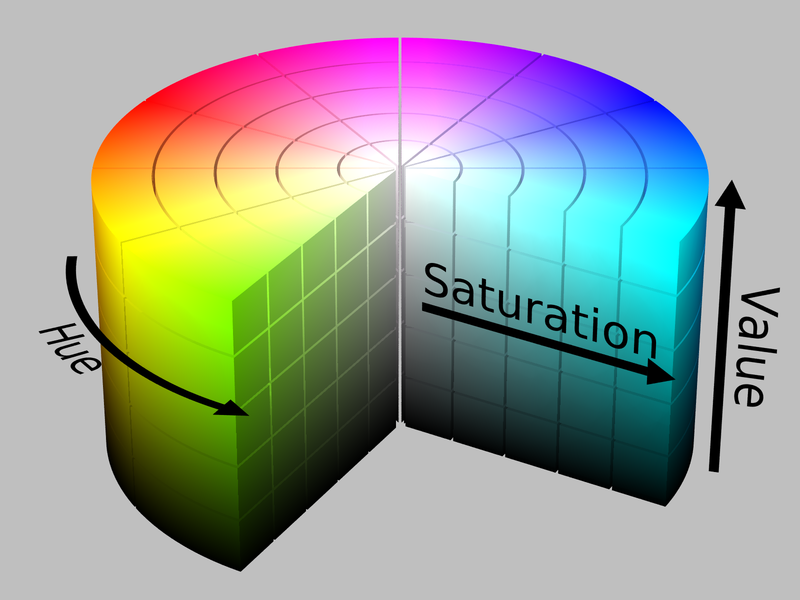# Hue

This is part of the WebGL image processing series and it relies on information in previous articles. See all articles here.

It is designed to be used on desktop.

## What is hue?

Hue is the main indication of a color. It's the value that defines the color itself.

Changing the hue of an image can change the color of the object it depicts, meaning a tomato would no longer be red, or that a banana would no longer be yellow.

HSB (hue, saturation, brightness) is an alternative to the RGB color model. In this model, colors of each hue are arranged in a radial slice around the central axis of neutral colors, with complete brightness at the top and darkness at the bottom.

Here are two diagrams from POV-Ray to illustrate the difference between RGB on the left, and HSV (or HSB) on the right.If we extract just the hue value, we can see how the color changes in the range of 0° to 360°. We have the red primary at 0°, the green primary at 120°, the blue primary at 240°, and then wrap back to red at 360°.

Hue color range from 0° to 360°

## Converting RGB to HSB

Similarly to how RGB values are mapped from 0 to 1 in GLSL instead of the common 0 to 255 range, we also need to adapt our HSB values. We want them to be mapped to 0 to 1, instead of 0° to 360°.

Hue color range from 0 to 1

In GLSL we can convert our RGB vec3 to a HSB vec3 with the following function:

vec3 rgb2hsb(vec3 c) {
vec4 K = vec4(0.0, -1.0 / 3.0, 2.0 / 3.0, -1.0);
vec4 p = mix(vec4(c.bg, K.wz), vec4(c.gb, K.xy), step(c.b, c.g));
vec4 q = mix(vec4(p.xyw, c.r), vec4(c.r, p.yzx), step(p.x, c.r));
float d = q.x - min(q.w, q.y);
float e = 1.0e-10;
return vec3(abs(q.z + (q.w - q.y) / (6.0 * d + e)), d / (q.x + e), q.x);
}


And back to RGB from HSB with the following function:

vec3 hsb2rgb(vec3 c){
vec4 K = vec4(1.0, 2.0 / 3.0, 1.0 / 3.0, 3.0);
vec3 p = abs(fract(c.xxx + K.xyz) * 6.0 - K.www);
return c.z * mix(K.xxx, clamp(p - K.xxx, 0.0, 1.0), c.y);
}


We'll use our standard photos (below) to see how these algorithms update an image's color values. The color gradient bar on the right is useful to see how individual color values change.

## Shifting an image's hue

We can shift the hue of our image easily once we convert our color values from an RGB color model into an HSB color model.

We can adjust the hue value to morph our image into another color. This means updating the first HSB value of our color.

void main() {
// map uv between 0 -> 1
vec2 uv = gl_FragCoord.xy/u_resolution;
vec4 texel = texture(u_image, uv);
outColor = texel;

vec3 hsb = rgb2hsb(texel.rgb);
hsb = 0.4;
outColor = vec4(hsb2rgb(hsb), 1.0);
}


Notice that the entire color range on the right is changed to a specific color hue. Let's look at how we can change only a specific range of hues.

## Shifting hue range

For this example, we'll break our HSB color range into 6 sections to enable us to pick a range to change.

Hue color range from 0 to 1
Hue color range broken into 6 sections

We've broken our 0 - 1 range into 6 sections. Red here would be 0 - 0.166, yellow 0.166 - 0.333 etc. This will give us some basic hue ranges to work with in our example below.

We can select our red range and then move the slider to change the hue. This will morph all the hues in this range into another hue. You'll see how a section of gradient on the right will change while the rest stays the same.

For the flower image, our first red range doesn't cover the entire red range of the flower itself. We also need some hues from the last magenta range.

But the green range manages to change all the green leaves in the background. This is up to luck, as the ranges were choosen by spliting our hue range into 6 sections.

We can achieve this effect in GLSL by only updating the hue if the texel's original hue value lies in-between our range's min and max values.

void main() {
vec3 hsb = rgb2hsb(texel.rgb);
if (hsb >= range_min && hsb <= range_max) {
hsb = u_fx;
}
outColor = vec4(hsb2rgb(hsb), 1.0);
}


In the next article, we'll look into the many ways we can blend two color values together.

## Feedback and suggestions

Have a suggestion or want to show me your work?
Get in touch at via email or Twitter @maximmcnair.